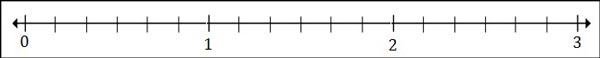# Plotting Fractions on a Number Line

A number line is a straight line with a 'zero' point in the middle, with positive and negative numbers marked on either side of zero and going on indefinitely. Here we plot fractions and mixed numbers on the given number line. The given number line has each of its units divided into a number of fractional parts. We identify the whole numbers between which the given fractional number lies and the fractional part it represents. Then we plot the given fraction on the given number line.

Plot 1$\frac{1}{2}$ and 2$\frac{4}{6}$ on the number line below### Solution

Step 1:

We must first count the number of parts between 0 and 1.

We find that the marks divide 1 unit into 6 equal parts. This means that the marks are 1/6 of a unit apart.

Step 2:

1$\frac{1}{2}$, lies between 1 and 2. Because 1$\frac{1}{2}$ = 1$\frac{3}{6}$. The point representing it is 3 marks to the right of 1.Step 3:

2$\frac{4}{6}$, lies between 2 and 3. The point representing it is 4 marks to the right of 2.

Plot 2$\frac{3}{8}$ and 1$\frac{1}{4}$ on the number line below### Solution

Step 1:

We find that the number line between 0 and 1 is divided into 8 parts.

Step 2:

We plot 1$\frac{1}{4}$ as 1$\frac{2}{8}$, two divisions after 1.

Step 3:

We plot 2$\frac{3}{8}$ as three divisions after 2.